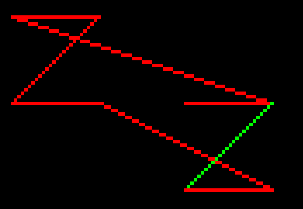# My Pixel Drawing Script Cant Draw A Cube And I Dont Know Why

Before i start i want to say i am using a Module script called CanvasDraw to draw pixels
So ive been trying to make a custom 3d rendering engine the past few hours and i ran into a bug where my script cant draw a cube heres what im talking aboutThe Green is the cubes orgin and i am using a table full of all the cubes points and i use a simple pair loop to go thorugh the all connecting them with the newest and last point i think it might be the placement of where i insert the vector2 vars im not too sure but heres my code

``````local GUI = script.Parent
local Frame = GUI.Canvas
local CanvasDraw = require(GUI.CanvasDraw)
local Canvas = CanvasDraw.new(Frame,Vector2.new(200, 100))
local orgin = Vector2.new(100,50)

function DrawLine(X,Y,Depth,Color,Table,Totalpoints)
local NewX = X-orgin.X/Depth
local NewY = Y-orgin.Y/Depth
Canvas:SetPixel(X,Y,Color)
table.insert(Table,Totalpoints,Vector2.new(X,Y))
Canvas:SetPixel(NewX,NewY,Color)
table.insert(Table,Totalpoints,Vector2.new(NewX,NewY))
end
function DrawCube(X,Y,Size,Depth,Color)
--Point placement
local points = {}
local totalpoints = 0
local CubeOrgin = nil
totalpoints+=1
DrawLine(X,Y,Depth,Color,points,totalpoints) X+=Size
CubeOrgin = Vector2.new(X,Y)
totalpoints+=1
DrawLine(X,Y,Depth,Color,points,totalpoints) X-=Size Y+=Size
totalpoints+=1
DrawLine(X,Y,Depth,Color,points,totalpoints) X+=Size
totalpoints+=1
DrawLine(X,Y,Depth,Color,points,totalpoints) X+=Size
--Line placement
local LastPoint = CubeOrgin
for _,Point in pairs(points) do
if Point == CubeOrgin then
Canvas:DrawLine(LastPoint,Point,Color3.new(0, 1, 0))
LastPoint = Point
else
Canvas:DrawLine(LastPoint,Point,Color)
LastPoint = Point
end
end
return points
end
DrawCube(100,50,25,2,Color3.new(1, 0, 0))
``````